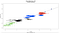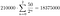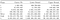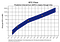# S2FX — Phase 5 Estimations

## WARNING: This is not financial advice. Entertainment purposes only.

In this article I will explore the regression identified by PlanB in his latest article. In particular, I will identify the prediction interval for the next halving cycle.

In PlanB’s article, he identifies four transitionary phases that hold in congruence to the different stock to flow ratios. The linear regression through the centre of these phases produces a line that intercepts both the gold and silver market caps providing some confidence that the asset is exiting an experimental phase and making a real economic impact.

As I am quite skeptical of these models, I will first reproduce the model using my own data driven technique (k means clustering), thus not relying on any assumptions from PlanBfigure 1 — log market cap for the four bitcoin phases (identified by k-means clustering) and log market cap of gold and silver versus log stock to flow.

Interestingly, the k-means algorithm finds almost the same relationship that PlanB identified. I will continue on using the relationship that k-means has identified.

In figure 1, the four phases are coloured green black blue and red, and the centres are given as points. The exponentiated data is provided for these centre points in table 1 below.

The regression summary is summarised in the table below.

The numbers in braces below the coefficient and constant are the standard errors of the coefficient (for those interested: to calculate the t statistic for the coefficient, divide the coefficient by the standard error. The t statistic is essentially the distance from zero). The standard error (SE) is the confidence we have in the coefficient. If the interval defined by the coefficient ± 1.96SE contains zero, then the coefficient is not significant. However; in this case the coefficient is a great number of standard errors away from zero (approximately 30SEs away from zero). Thus, the coefficient is statistically significant at at least the 0.01 level (and in reality when we actually look this value up from the t distribution it is functionally equivelant to zero). This means, the chance of the coefficient being this number instead of zero by chance is almost nil.

The R² is very high, and whilst this can be interesting it is not something to focus on — the point to remember here is that there is a very high correlation between the stock to flow cluster centres and the market cap centre clusters. Keen students will notice that whilst the number of observations are small, the F statistic has been adjusted for the appropriate degrees of freedom (df). The F statistic tells us that the chance of the coefficient and the constant simultaneously being zero and us getting this result is also almost nil.

I will leave the exploration of the Gauss-Markov assumptions for a future article, but given the number of data points presently, I suspect it will be a rather dull exercise.

Now, let us work out the prediction interval. First, lets define what that is (and contrast it to a confidence interval).

A confidence interval is an interval which contains an unknown characteristic of the sampled population or process. These usually relate to parameters like means or variances etc.

A confidence interval captures the uncertainty around the mean predicted values.

A prediction interval is an interval which contains one or more future observations, or some function of such future observations, from a previously sampled population or process.

A prediction interval captures the uncertainty around a single value.

Thus, a prediction interval will always be wider than a confidence interval for the same value.

Think of a confidence interval as the confidence in the line, whereas a prediction interval is like the confidence of an individual prediction.

BUT before we do this, we need to estimate the centre of the next phase. Thanks to Satoshi, this is very easy. We know that every 210000 blocks the halving occurs.We know the initial reward was 50 coins. Ergo, we can formulate:equation 1 — calculation for number of bitcoin at the 3rd halving

Thus there will be a stock of 18375000 bitcoins. By the fourth halving, this will have increased to 19687500. Thus, we get a flow of 19687500–18375000 =1312500 bitcoins during the period of 210000 blocks. To annualise this into the correct format for stock to flow, we multiply by the number of days per block. i.e flow = 1312500 * (6*24*365.25/210000) = 328725 coins per year, on average for the halving period. This gives us a stock to flow ratio at the fourth halving of 19687500/328725 = 59.9. Taking the natural log, we get 4.09.table 3 — boundary conditions of the market cap for the different clustering phases

The boundary for the fifth phase is estimated at between 1.6 trillion and 29.1 trillion USD. Assuming the end of the phase market evaluation, this translates to an individual bitcoin price of between \$83k and \$1.48m (with a centre point of around 350k).

Don’t forget to catch me on twitter: https://twitter.com/btconometrics and visit my website at https://btconometrics.comFigure 2 — Transposing the data in table 3 to a time series graph, we can see the power of the model.

Nick.

Source file for the above work available here: https://btconometrics.com/cluster.R

## References:

@PlanB 2020 Bitcoin Stock-to-Flow Cross Asset Model https://medium.com/@100trillionUSD/bitcoin-stock-to-flow-cross-asset-model-50d260feed12

Forgy, E. W. (1965). Cluster analysis of multivariate data: efficiency vs interpretability of classifications. Biometrics, 21, 768–769.

Hartigan, J. A. and Wong, M. A. (1979). Algorithm AS 136: A K-means clustering algorithm. Applied Statistics, 28, 100–108. doi: 10.2307/2346830.

Lloyd, S. P. (1957, 1982). Least squares quantization in PCM. Technical Note, Bell Laboratories. Published in 1982 in IEEE Transactions on Information Theory, 28, 128–137.

MacQueen, J. (1967). Some methods for classification and analysis of multivariate observations. In Proceedings of the Fifth Berkeley Symposium on Mathematical Statistics and Probability, eds L. M. Le Cam & J. Neyman, 1, pp. 281–297. Berkeley, CA: University of California Press.

Hlavac, Marek (2018). stargazer: Well-Formatted Regression and Summary Statistics Tables. R package version 5.2.2. https://CRAN.R-project.org/package=stargazer

Get Best Software Deals Directly In Your Inbox

## Coinmonks

### By Coinmonks

A newsletter that brings you week's best crypto and blockchain stories and trending news directly in your inbox, by CoinCodeCap.com Take a look

Written by

Written by

## phraudsta

#### https://www.btconometrics.com/